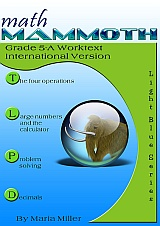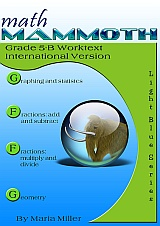THANKSGIVING sale! Get 25% off of all of Math Mammoth and Make It Real Learning products sold on this site. Use coupon code V4PN-JSZA-V42F. The offer is valid till December 1, 2019.

^
You are here: HomeMM International Version → Grade 5

# Math Mammoth Grade 5 International Version

Math Mammoth Grade 5 International Version is a complete maths program for fifth grade. It consists of two student worktexts (A and B), a separate answer key for each, tests, mixed revisions, and in the download version, an additional worksheet maker (Internet access required) to make extra practice worksheets when needed.205 pages
170 lesson pages196 pages
176 lesson pages

## Prices & ordering

(part A and B worktexts, answer keys,
tests, mixed revisions, worksheet
maker, and Soft-Pak)

Part 5-A only: \$18.75
(part A worktext, part A answer key,
tests, mixed revisions, worksheet
maker, and Soft-Pak)

Part 5-B only: \$18.75
(part B worktext, part B answer key,
tests, mixed revisions, worksheet
maker, and Soft-Pak)

#### Printed copies

You can purchase printed hardcopies at Lulu. The prices below are in USD.

5-A worktext \$16.95
5-B worktext \$16.95
Tests & Mixed Revisions \$12.45

This curriculum is essentially the same as the US version of Math Mammoth Grade 5. The program is customised for the international audience in these aspects:

• The curriculum teaches the metric measurement units. Imperial units, such as inches and pounds, are not used.
• The spelling conforms to British international standards (British English).
• Page (paper) size is A4.
• Large numbers are formatted with a space as the thousands separator (such as 12 394). (The decimals are formatted with a decimal point, like in the US version.)

In money-related word problems, the symbol it uses is "\$" (dollar).

## Introduction

The fifth grade is time for fractions and decimals, in particular. In part 5-A, we study decimals in depth and with substantial detail. Students also deepen their understanding of whole numbers, learn much more problem solving, and are introduced to the calculator.

The year starts out with a study of whole numbers, their operations, and problem solving. Students get to revise multi-digit multiplication and long division. Then we study equations with the help of a balance and bar models (simple diagrams). The main idea is to get students used to the idea of an equation and what it means to solve an equation.

In the second chapter, the focus is on large numbers and using a calculator. This is the first time a calculator is introduced in Math Mammoth complete curriculum — so far, all calculations have been done mentally, or with paper and pencil. I want students to learn to be careful in their use of the calculator — use it with good judgment. Every exercise where calculator use is to be allowed is marked with a symbol of a small calculator.

The fourth chapter is about decimals and their operations. It is a long chapter because now is the time to learn decimal operations well. It is assumed that the student already has a solid foundation for decimal place value, as taught in Math Mammoth Grade 4 curriculum. That is the true means of preventing common misconceptions, or students resorting to rote memorisation of the decimal operations.

In chapter 5, we study graphing in a coordinate grid, line and bar graphs, and average and mode. Today's world has become increasingly complex, with lots of data in the media, so our children need a good grasp of graphs to be able to make sense of all that information.

Chapter 6 is about the addition and subtraction of fractions — another focus topic for 5th grade, besides decimals. Students learn to add and subtract unlike fractions, using the technique of first converting them to equivalent like fractions. In chapter 7, we study the multiplication and division of fractions (division only in special cases), relying first on visual models, and then proceeding to the abstract shortcuts.

Chapter 8 takes us to geometry, starting with a revision of angles and polygons. From there, students will learn to draw circles, to classify triangles and quadrilaterals, and the concept of volume in the context of right rectangular prisms (boxes).

Please also see the table of contents for 5-A and 5-B (in the sample), which will let you see the topics covered in more detail.

Some main features of Math Mammoth complete curriculum (Light Blue series) are:

• focuses on understanding of mathematical concepts
• uses clear explanations, lots of visual exercises and pattern exercises
• mastery oriented: concentrates at length on a topic, with very few topics per grade
• emphasizes mental maths and developing number sense
• very little teacher preparation needed

The downloadable version includes a worksheet maker (Internet access required) that lets you make extra practice worksheets for many of the topics in the curriculum.

The PDFs in the download version are enabled for annotation. This means that if you prefer, the student can fill them in on the computer, using the typewriter and drawing tools in Acrobat Reader version 9 or greater.

## Manipulatives

Here is a list of manipulatives that are needed or recommended for grade 5.

1. A ruler that measures in centimetres (for 5-B).
2. A compass and protractor (for 5-B).
3. Fraction manipulatives. The curriculum contains printables to make your own fraction manipulatives. The text uses visual pie models extensively, and students even learn to draw them themselves for the exercises, therefore making or buying the manipulatives is entirely optional (optional; for 5-B).

## How and where to order

You can buy Math Mammoth International Version:

• On this site (Mathmammoth.com) — using the "Add to cart" buttons.
• At Currclick.

By purchasing any of the books, permission IS granted for the purchaser (teacher or parent) to reproduce this material to be used with his/her students in a teaching situation; not for commercial resale. Please note: you are not permitted to share the material with other teachers or parents.

In other words, you are permitted to make copies for the students/children you are teaching, but not for other teachers' usage.

WAIT!

Receive my monthly collection of math tips & resources directly in your inbox — and get a FREE Math Mammoth book!You can unsubscribe at any time.

### Math Mammoth Tour

Confused about the different options? Take a virtual email tour around Math Mammoth! You'll receive:

An initial email to download your GIFT of over 400 free worksheets and sample pages from my books. Six other "TOURSTOP" emails that explain the important things and commonly asked questions concerning Math Mammoth curriculum. (Find out the differences between all these different-colored series!)

This way, you'll have time to digest the information over one or two weeks, plus an opportunity to ask me personally about the curriculum.
A monthly collection of math teaching tips & Math Mammoth updates (unsubscribe any time)### "Mini" Math Teaching Course

This is a little "virtual" 2-week course, where you will receive emails on important topics on teaching math, including:

- How to help a student who is behind
- Troubles with word problems
- Teaching multiplication tables
- Why fractions are so difficult
- The value of mistakes
- Should you use timed tests
- And more!

A GIFT of over 400 free worksheets and sample pages from my books right in the very beginning.A monthly collection of math teaching tips & Math Mammoth updates (unsubscribe any time)
Enter your email to receive math teaching tips, resources, Math Mammoth news & sales, humor, and more! I tend to send out these tips about once monthly, near the beginning of the month, but occasionally you may hear from me twice per month (and sometimes less often).• A GIFT of over 400 free worksheets and sample pages from my books.